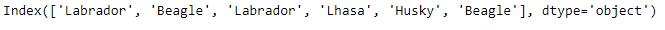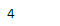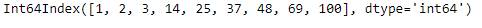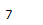# Python | Pandas Index.get_slice_bound()

• Last Updated : 17 Dec, 2018

Python is a great language for doing data analysis, primarily because of the fantastic ecosystem of data-centric python packages. Pandas is one of those packages and makes importing and analyzing data much easier.

Pandas` Index.get_slice_bound()` function calculate slice bound that corresponds to given label and it returns the value. The function returns the leftmost position of the given label if side parameter is set to be left and it returns one-past the rightmost position of the label if side parameter is set to be right.

Attention geek! Strengthen your foundations with the Python Programming Foundation Course and learn the basics.

To begin with, your interview preparations Enhance your Data Structures concepts with the Python DS Course. And to begin with your Machine Learning Journey, join the Machine Learning - Basic Level Course

Syntax: Index.get_slice_bound(label, side, kind)

Parameters:
label : object
side : {‘left’, ‘right’}
kind : {‘ix’, ‘loc’, ‘getitem’}

Returns : value of the bound

Example #1: Use `Index.get_slice_bound()` function to find the right slice bound of the passed value.

 `# importing pandas as pd``import` `pandas as pd`` ` `# Creating the Index``idx ``=` `pd.Index([``'Labrador'``, ``'Beagle'``, ``'Labrador'``,``                     ``'Lhasa'``, ``'Husky'``, ``'Beagle'``])`` ` `# Print the Index``idx`

Output :let’s find out the slice bound of the passed value.

 `# Print the right slice bound of the passed value..``idx.get_slice_bound(``'Lhasa'``, side ``=``'right'``, kind ``=``'getitem'``)`

Output :As we can see in the output, the `Index.get_slice_bound()` function has returned 4 as this is position one past the position of the passed value in the Index.

Example #2: Use `Index.get_slice_bound()` function to find the left slice bound of the passed value.

 `# importing pandas as pd``import` `pandas as pd`` ` `# Creating the Index``idx ``=` `pd.Index([``1``, ``2``, ``3``, ``14``, ``25``, ``37``, ``48``, ``69``, ``100``])`` ` `# Print the Index``idx`

Output :Let’s find the left slice bound of the value 69 in the Index.

 `# Find the left slice bound of 69 in the Index.``idx.get_slice_bound(``69``, side ``=``'left'``, kind ``=``'getitem'``)`

Output :As we can see the function has returned the output 7 as this is the position of the passed value and that of the left slice bound for the passed value.

My Personal Notes arrow_drop_up